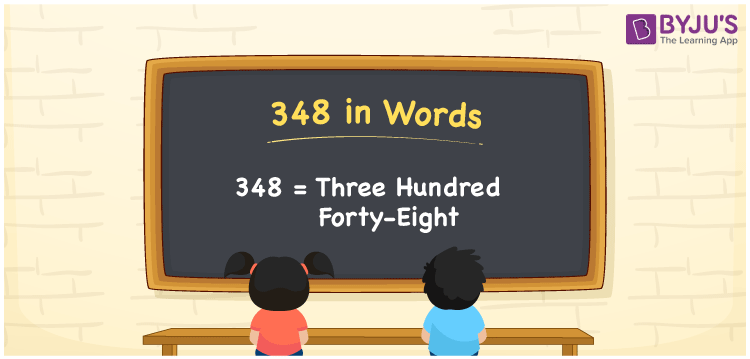# 348 in Words

348 in words is written as Three hundred forty-eight. In both the International System of Numerals and the Indian System of Numerals, 348 is written as Three hundred forty-eight. The number 348 is a Cardinal Number as it represents some quantity. For example, “there are 348 chairs in the room”.

 348 in Words Three hundred forty-eight Three hundred forty-eight in Number 348

## 348 in English Words

We write 348 in English Words using the letters of the English alphabet. Therefore, we read 348 in English as “Three hundred forty-eight.”## How to Write 348 in Words?

To write 348 in words, we shall use the place value chart. In the place value chart, write 3 in the hundreds, 4 in the tens, and 8 in the ones, respectively. Now let us make a place value chart to write the number 348 in words.

 Hundreds Tens Ones 3 4 8

Thus, we can write the expanded form as

3 × Hundred + 4 × Ten + 8 × One

= 3 × 100 + 4 × 10 + 8 × 1

= 300 + 40 + 8

= 348

= Three hundred forty-eight.

348 is a natural number, the successor of 347 and the predecessor of 349.

348 in words – Three hundred forty-eight

• Is 348 an odd number? – No
• Is 348 an even number? – Yes
• Is 348 a perfect square number? – No
• Is 348 a perfect cube number? – No
• Is 348 a prime number? – No
• Is 348 a composite number? – Yes

## Frequently Asked Questions on 348 in Words

Q1

### How to write 348 in words?

348 in words is written as Three hundred forty-eight.
Q2

### How to write 348 in the International and Indian System of Numerals?

In both, the system of numerals, 348 in words, is written as Three hundred forty-eight.
Q3

### What is the preceding number of 348?

The number that precedes 348 is 347.